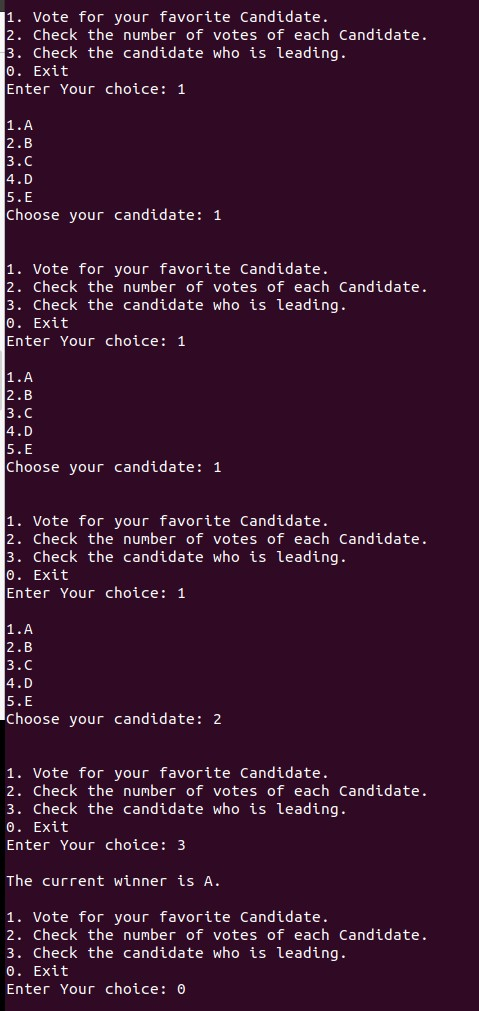# Menu driven program for Voting System

• Last Updated : 18 Mar, 2021

In this article, we will write a menu-driven program to implement the Voting System. The program must contain the following properties:

• Display the count of votes of each candidate.
• Display the name of the candidate who has the most votes.

Approach: Follow the steps below to solve the problem:

1. Provide the following options to the person who is accessing as shown below:
• Vote for your favorite Candidate.
• Check the number of votes of each Candidate.
• Check the candidate who is leading and then Exit.
2. The user chooses one of the options.
3. If the user chooses 1, then the list of candidates is displayed and the user can now choose from this list of candidates.
4. If the user chooses 2, then the list of candidates along with their current number of votes is displayed.
5. If the user chooses 3, the name of the candidate with the maximum number of votes is displayed. If there is more than one candidate with maximum votes, display an error message stating “No winner”.
6. This program continues until the user chooses 0 to exit().

Below is the implementation of the above approach:

## C++

 `// C++ program for the above approach``#include ``using` `namespace` `std;`` ` `// Driver Code``int` `main()``{``    ``int` `choice, i, N;`` ` `    ``// Stores the names of candidates``    ``vector candidates``        ``= { ``"A"``, ``"B"``, ``"C"``, ``"D"``, ``"E"` `};``    ``N = candidates.size();`` ` `    ``// Stores the votes of candidates``    ``vector<``int``> votes(N);``    ``do` `{``        ``cout << ``"\n1. Vote for your "``             ``<< ``"favorite Candidate.\n"``;``        ``cout << ``"2. Check the number "``             ``<< ``"of votes of each "``                ``"Candidate.\n"``;``        ``cout << ``"3. Check the candidate "``             ``<< ``"who is leading.\n"``;``        ``cout << ``"0. Exit\n"``;`` ` `        ``// Take input of options``        ``cout << ``"Enter Your choice: "``;``        ``cin >> choice;``        ``cout << ``"\n"``;`` ` `        ``// Switch Statement``        ``switch` `(choice) {`` ` `        ``case` `1: {``            ``int` `candidatechoice;`` ` `            ``// Display the names of``            ``// all the candidates``            ``for` `(i = 0; i < N; i++)``                ``cout << i + 1 << ``"."``                     ``<< candidates[i]``                     ``<< ``"\n"``;`` ` `            ``cout << ``"Choose your candidate: "``;`` ` `            ``// Taking user's vote``            ``cin >> candidatechoice;``            ``cout << ``"\n"``;`` ` `            ``// Update the vote of the``            ``// chosen candidate``            ``votes[candidatechoice - 1]++;``            ``break``;``        ``}``        ``case` `2: {`` ` `            ``// Display the name and votes``            ``// of each``            ``// candidate``            ``for` `(i = 0; i < N; i++)``                ``cout << i + 1 << ``"."``                     ``<< candidates[i] << ``" "``                     ``<< votes[i] << ``"\n"``;``            ``break``;``        ``}``        ``case` `3: {``            ``int` `mx = 0;``            ``string winner;`` ` `            ``// Find the candidate with``            ``// maximum votes``            ``for` `(``int` `i = 0; i < N; i++)``                ``if` `(votes[i] > mx) {``                    ``mx = votes[i];``                    ``winner = candidates[i];``                ``}``            ``int` `flag = 0;`` ` `            ``// Check whether there are``            ``// more than one candidates``            ``// with maximum votes``            ``for` `(``int` `i = 0; i < N; i``                  ` `                ``if` `(votes[i] == mx``                    ``&& winner != candidates[i]) {``                ``flag = 1;``                ``break``;``                ``}``            ``if` `(!flag)``                ``cout << ``"The current winner is "``                 ``<< winner    << ``".\n"``;``            ``else``                ``cout << ``"No clear winner\n"``;``        ``}``        ``default``:``            ``"Select a correct option"``;``        ``}``    ``} ``while` `(choice != 0);`` ` `    ``return` `0;``}`

Output:My Personal Notes arrow_drop_up